# Distance Midpoint Pythagorean Theorem Distance Formula Distance formulaused

• Slides: 17Distance, Midpoint, Pythagorean Theorem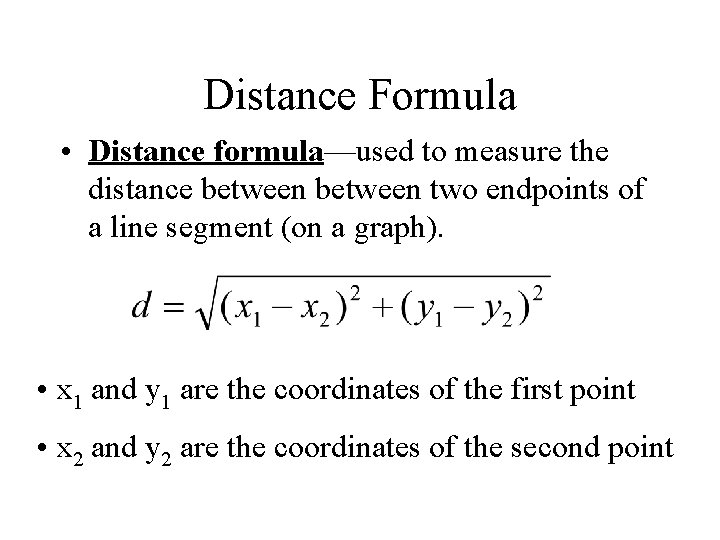Distance Formula • Distance formula—used to measure the distance between two endpoints of a line segment (on a graph). • x 1 and y 1 are the coordinates of the first point • x 2 and y 2 are the coordinates of the second pointDistance Formula • The distance between two points A(x 1 , y 1) and B(x 1 , y 1) isFinding Distance • What is the distance between U(-7 , 5) and V(4 , -3)? Round to the nearest tenth?Distance Formula examples • Find the distance between the points (1, 2) and (– 2, – 2). • Your video game uses a coordinate grid system for location. There is an enemy ship at (7, – 3). You are at (– 8, – 3). If one grid unit equals 10 miles, how far away is the enemy ship?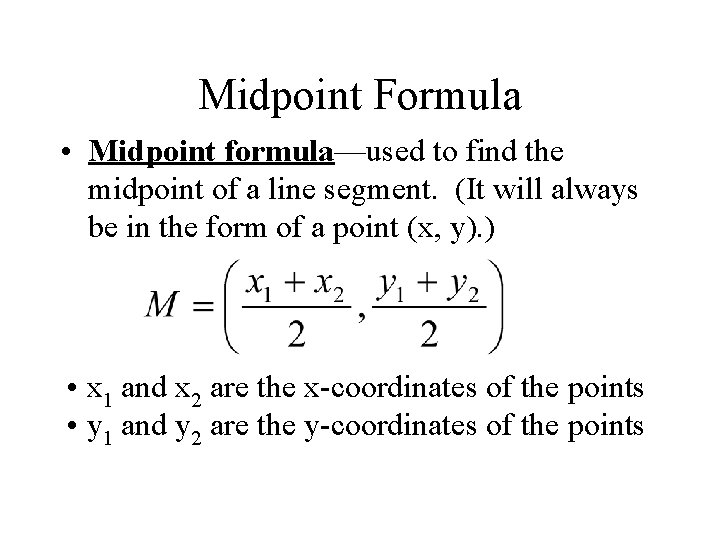Midpoint Formula • Midpoint formula—used to find the midpoint of a line segment. (It will always be in the form of a point (x, y). ) • x 1 and x 2 are the x-coordinates of the points • y 1 and y 2 are the y-coordinates of the pointsFinding the Midpoint • Segment AB has endpoints at -4 and 9. What is the coordinate of its midpoint?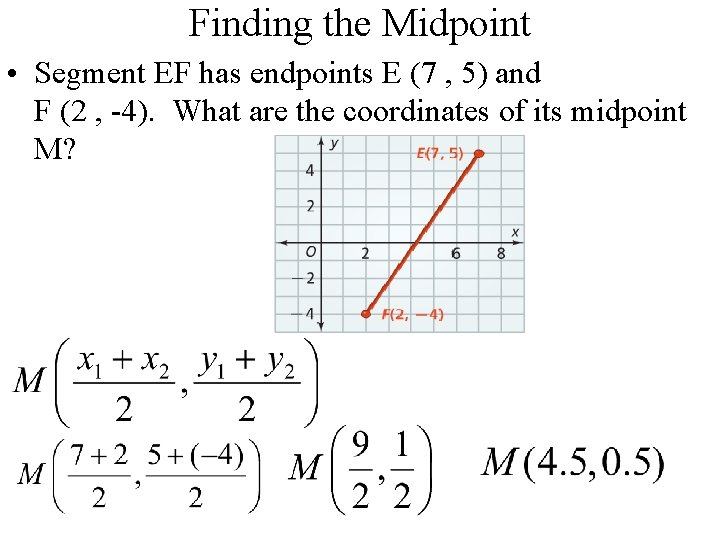Finding the Midpoint • Segment EF has endpoints E (7 , 5) and F (2 , -4). What are the coordinates of its midpoint M?Finding an Endpoint • The midpoint of segment CD is M(-2 , 1). One endpoint is C (-5 , 7). What are the coordinates if the other endpoint D?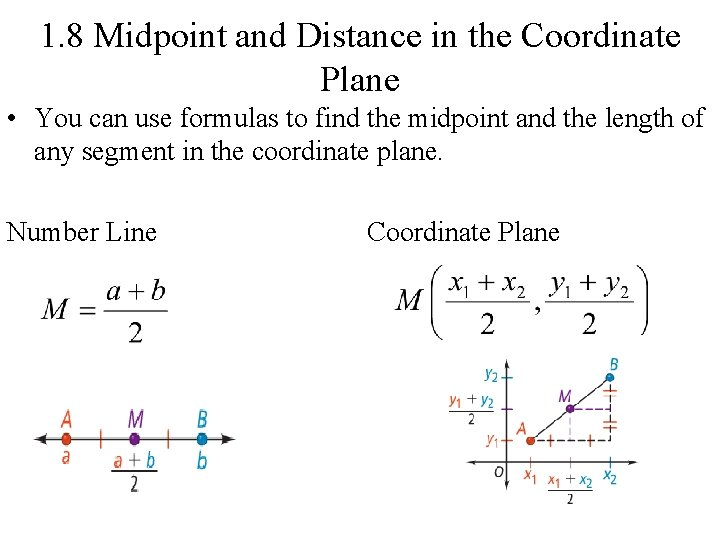1. 8 Midpoint and Distance in the Coordinate Plane • You can use formulas to find the midpoint and the length of any segment in the coordinate plane. Number Line Coordinate Plane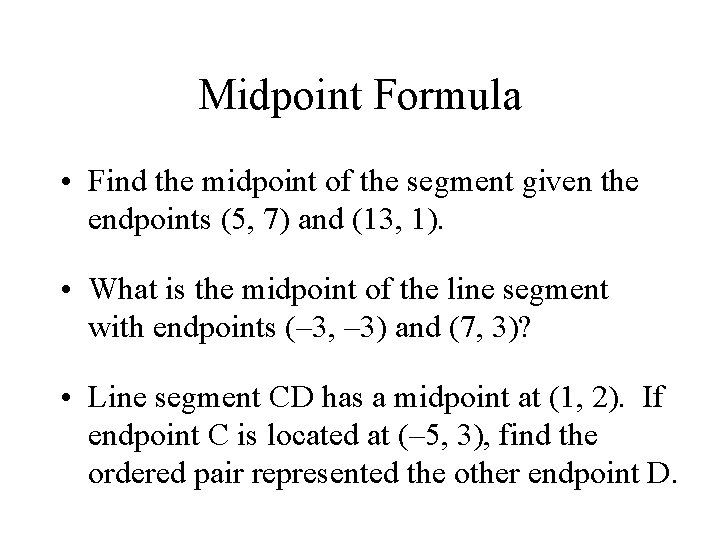Midpoint Formula • Find the midpoint of the segment given the endpoints (5, 7) and (13, 1). • What is the midpoint of the line segment with endpoints (– 3, – 3) and (7, 3)? • Line segment CD has a midpoint at (1, 2). If endpoint C is located at (– 5, 3), find the ordered pair represented the other endpoint D.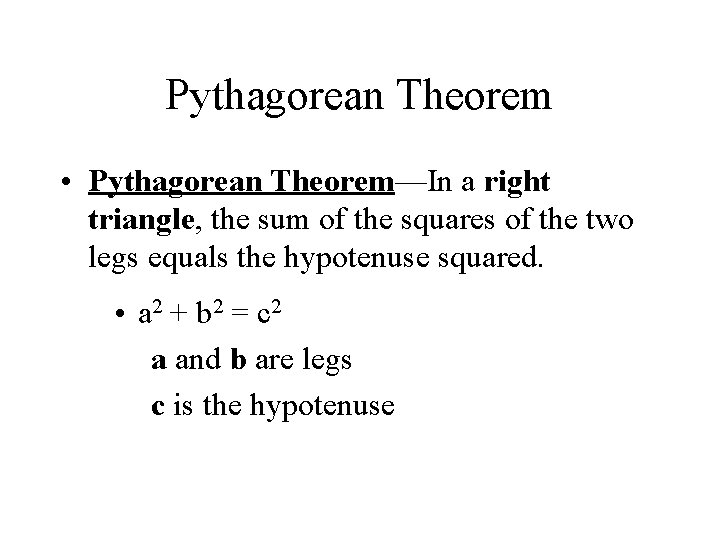Pythagorean Theorem • Pythagorean Theorem—In a right triangle, the sum of the squares of the two legs equals the hypotenuse squared. • a 2 + b 2 = c 2 a and b are legs c is the hypotenusePythagorean Theorem hypotenuse leg The hypotenuse is always the longest side of a right triangle and is always opposite the right angle.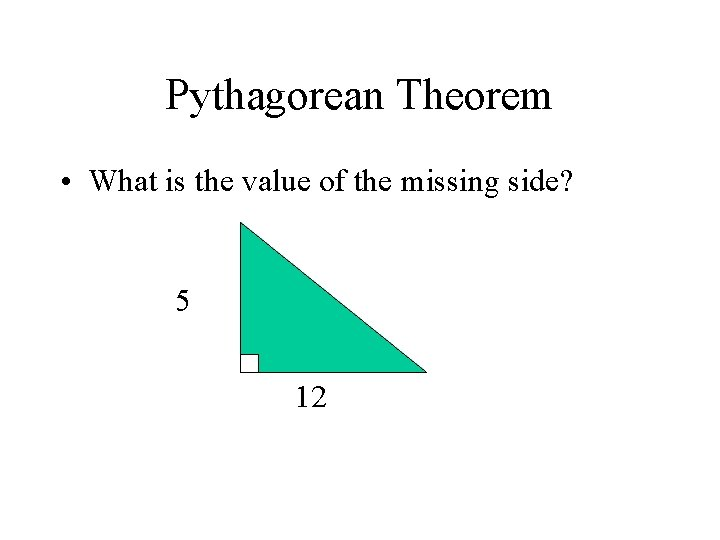Pythagorean Theorem • What is the value of the missing side? 5 12Pythagorean Theorem • What is the value of the missing side? 9 15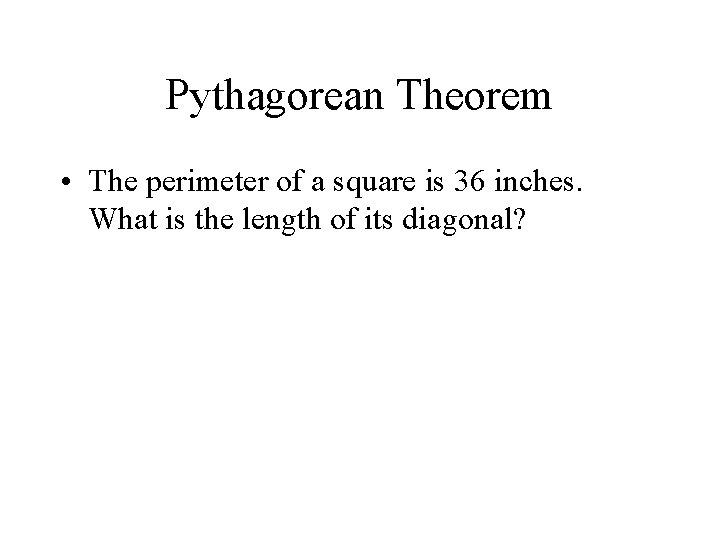Pythagorean Theorem • The perimeter of a square is 36 inches. What is the length of its diagonal?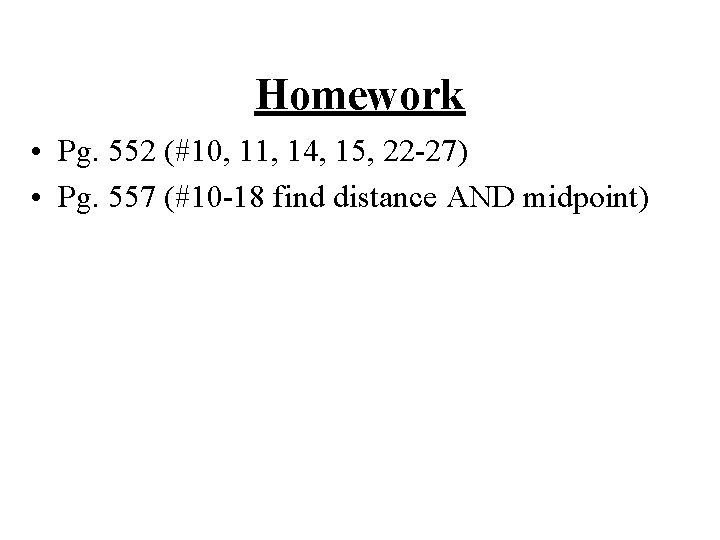Homework • Pg. 552 (#10, 11, 14, 15, 22 -27) • Pg. 557 (#10 -18 find distance AND midpoint)